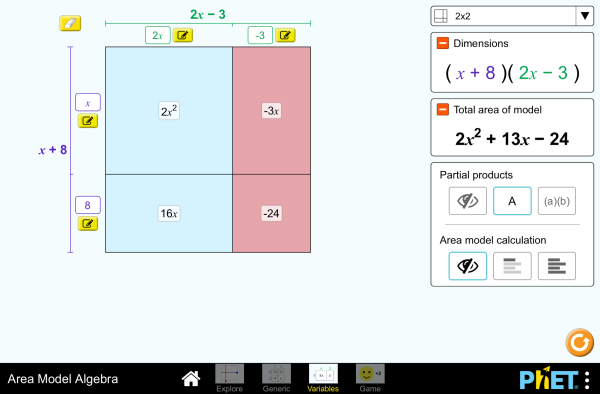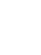# වර්ගඵල ආකෘති වීජ ගණිතයබාගත කරන්නඔබ්බනවා වසන්න මෙම අනුහුරුකරණයෙහි ක්‍රියාත්මක පිටපතක් කාවද්දන්න. මෙම අනුහුරුකරණයෙහි ක්‍රියාත්මක පිටපතක් කාවැද්දීමට මෙම HTML භාවිත කරන්න. HTML වල ඇති ඇති"width" සහ "height" යන HTML හි ගුණාංග වෙනස් කරමින් .ඔබ කාවැද්දූ අනුහුරුකරණයෙහි පළල සහ උස වෙනස් කල හැකිය. මත ක්ලික් කරන විට අනුහුරුකරණ දියත් කරන රූපයක් කාවද්දන්න
ක්‍රියාත්මක කිරීමට ක්ලික් කරන්න
"Click to Run" වචන සමග තිර පිටපතක් පෙන්වීම සදහා මෙම HTML කේතය භාවිතා කරන්න බහුපද Factors නිෂ්පාදන PhET සහය ලබන්නේසහ ඔබවැනි අධ්‍යාපනඥයින්

• බහුපද
• Factors
• නිෂ්පාදන

### විස්තර

Build rectangles of various sizes and relate multiplication to area. Discover new strategies for multiplying algebraic expressions. Use the game screen to test your multiplication and factoring skills!

### ආදර්ශ ඉගෙනුම් ඉලක්ක

• Develop and justify a method to use the area model to determine the product of a monomial and a binomial or the product of two binomials.
• Factor an expression, including expressions containing a variable.
• Recognize that area represents the product of two numbers and is additive.
• Represent a multiplication problem as the area of a rectangle, proportionally or using generic area.
• Develop and justify a strategy to determine the product of two multi-digit numbers by representing the product as an area or the sum of areas.

### Standards Alignment

#### Common Core - Math

3.MD.C.7
Relate area to the operations of multiplication and addition.
3.MD.C.7c
Use tiling to show in a concrete case that the area of a rectangle with whole-number side lengths a and b + c is the sum of a × b and a × c. Use area models to represent the distributive property in mathematical reasoning.
3.MD.C.7d
Recognize area as additive. Find areas of rectilinear figures by decomposing them into non-overlapping rectangles and adding the areas of the non-overlapping parts, applying this technique to solve real world problems.
4.NBT.B.5
Multiply a whole number of up to four digits by a one-digit whole number, and multiply two two-digit numbers, using strategies based on place value and the properties of operations. Illustrate and explain the calculation by using equations, rectangular arrays, and/or area models.
6.EE.A.3
Apply the properties of operations to generate equivalent expressions. For example, apply the distributive property to the expression 3 (2 + x) to produce the equivalent expression 6 + 3x; apply the distributive property to the expression 24x + 18y to produce the equivalent expression 6 (4x + 3y); apply properties of operations to y + y + y to produce the equivalent expression 3y.
6.EE.A.4
Identify when two expressions are equivalent (i.e., when the two expressions name the same number regardless of which value is substituted into them). For example, the expressions y + y + y and 3y are equivalent because they name the same number regardless of which number y stands for..
6.NS.B.4
Find the greatest common factor of two whole numbers less than or equal to 100 and the least common multiple of two whole numbers less than or equal to 12. Use the distributive property to express a sum of two whole numbers 1-100 with a common factor as a multiple of a sum of two whole numbers with no common factor. For example, express 36 + 8 as 4 (9 + 2)..
7.EE.A.1
Apply properties of operations as strategies to add, subtract, factor, and expand linear expressions with rational coefficients.
7.NS.A.2c
Apply properties of operations as strategies to multiply and divide rational numbers.
8.EE.C.7b
Solve linear equations with rational number coefficients, including equations whose solutions require expanding expressions using the distributive property and collecting like terms.
අනුවාදය 1.2.1

### ගුරුවරුන්ට ඉඟිගුරු මාර්ගෝපදේශ සංග්‍රහයෙහි (pdf) PhET කණ්ඩායම විසින් නිර්මාණය කරන ලද ඉඟි අඩංගු වේ. ( පීඩීඑෆ් ).

### වීඩියෝ ප්‍රාරම්භය

වීඩියෝ ප්‍රාරම්භය නැරඹීමට කරුණාකර පිවිසෙන්න

### ගුරුවරුන් ඉදිරිපත් කල ක්‍රියාකාරකම්

Games Remote Lesson ideasTrish Loeblein HS
K-5
MS
UG-Intro
HW
Remote
Physics
Chemistry
Mathematics
Expanding and Factoring Algebraic ExpressionsAmanda McGarry MS Guided Mathematics
Algebraic ExpressionsAmanda McGarry MS Guided Mathematics
Exploring Factoring as Undoing the Distributive PropertySarah Hampton HS
MS
Guided Mathematics
Middle School Math Sim AlignmentAmanda McGarry MS Other Mathematics
Using the area model with expressionsJennifer Knudsen, Teresa Lara-Meloy, Amanda McGarry MS Discuss Mathematics
SECUNDARIA: Alineación PhET con programas de la SEP México (2011 y 2017)Diana López MS
HS
Other Biology
Chemistry
Mathematics
Physics
PREPARATORIA: Alineación de PhET con programas de la DGB México (2017)Diana López HS
UG-Intro
Other Physics
Mathematics
Chemistry
Would You Rather ... Karen Gann HS Guided Mathematics
Area Model Algebra - Multiplying Polynomials Carolyn Felknor MS Guided Mathematics
ARTICULANDO ÁLGEBRA E GEOMETRIA: O CASO DAS EQUAÇÕES ALGÉBRICAS NO ESTUDO DE ÁREAS Verusca Batista Alves; Ana Carolina Costa Pereira MS Lab
HW
Mathematics
Distributive Property Review Tim Kissinger and Larry Peters MS Guided
Discuss
Mathematics
Modelo de Áreas, Cambio de Expresiones Andrés Gualberto Palomo Cuevas HS Remote
HW
Mathematics
භාෂාව Downloador RunTips
Albanian All shqipModeli Syprinës: Algjebër
Basque All EuskaraAzalera-modeloan algebra
Chinese (China) All 中文 (中国)面积模型代数
Chinese (Taiwan) All 中文 (台灣)Area Model Algebra_面積模型與代數
Dutch All NederlandsAlgebra van oppervlakte
Finnish All suomiPinta-ala ja algebra
French All françaisModélisation par les aires - Algèbre
Gujarati All Gujaratiબીજગણિતનું ક્ષેત્રફળ મોડેલ -નિકુંજ સવાણી
Hindi All हिंदीबीजगणित -नमूने का क्षेत्रफल
Hungarian All magyarTerületmodell: algebra
Italian All italianoRappresentazione grafica della moltiplicazione in algebra
Japanese All 日本語面積と式の展開
Kazakh All KazakhАуданды есептеу
Korean All 한국어면적 모형: 대수
Latvian All LatviešuLaukuma modeļa algebra
Mongolian All Монгол (Монгол)Талбайн загварын Алгебр
Persian All فارسیجبر مدل مساحت
Polish All polskiModel pola - wyrażenia algebraiczne
Portuguese All portuguêsModelo de Área: Álgebra
Portuguese (Brazil) All português (Brasil)Modelo de Área: Álgebra
Russian All русскийВычисление площади
Serbian All СрпскиМатематика преко површина - Алгебра
Swedish All svenskaAreamodellen och algebra
Tamil All Tamilமாதிரி உருவின் எண்(அட்சர) கணிதம்
Turkish All TürkçeAlan Modeli İle Cebir
Ukrainian All українськаПлощинна модель. АлгебраVietnamese All Tiếng ViệtĐại số với mô hình diện tích
අරාබි All العربيةجبر نموذج المساحة
ඉංග්‍රීසි All EnglishArea Model Algebra
ග්‍රීක් All ΕλληνικάΥπολογισμός εμβαδού
ජර්මන් All DeutschFlächenmodell Algebra
ඩැනිෂ් All DanskArealmodel, algebra
බොස්නියානු All BosanskiMatematički model površina - Algebra
ස්පැනිෂ් All españolModelo de Áreas: Álgebraස්පැනිෂ්(පේරු) All español (Perú)Modelo de Área con Álgebra
ස්පැනිෂ්(මෙක්සිකෝ) All español (México)Modelo de Áreas: ÁlgebraHTML5 sims can run on iPads and Chromebooks, as well as PC, Mac, and Linux systems.

iOS 12+ Safari

Android:
Not officially supported. If you are using the HTML5 sims on Android, we recommend using the latest version of Google Chrome.

Chromebook:
The HTML5 and Flash PhET sims are supported on all Chromebooks.
Chromebook compatible sims

Windows Systems:

Macintosh Systems:

Linux Systems:

නිර්මාණ කණ්ඩායම තෙවන පාර්ශවීය පුස්තකාල අපගේ ස්තුතිය
• Jonathan Olson (developer)
• Karina Hensberry
• Susan Miller
• Ariel Paul
• Kathy Perkins
• Mariah Hermsmeyer (artwork)
• Diana López Tavares (artwork)
• almond-0.2.9.js
• base64-js-1.2.0.js
• FileSaver-b8054a2.js
• font-awesome-4.5.0
• game-up-camera-1.0.0.js
• he-1.1.1.js
• himalaya-0.2.7.js
• jama-1.0.2
• jquery-2.1.0.js
• lodash-4.17.4.js
• pegjs-0.7.0.js
• seedrandom-2.4.2.js
• text-2.0.12.js
• TextEncoderLite-3c9f6f0.js